## Linearly Dependent Functions

Thefunctions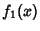,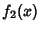, ...,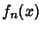are linearly dependent if, for some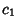,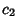, ...,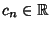not all zero,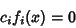(1)

(where Einstein Summation is used) for allin some interval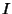. If the functions are not linearly dependent, they are said to be linearly independent. Now, if the functions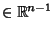, we can differentiate (1) up to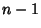times. Therefore, linear dependence also requires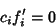(2)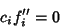(3)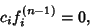(4)

where the sums are over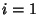, ...,. These equations have a nontrivial solution Iff the Determinant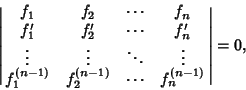(5)

where the Determinant is conventionally called the Wronskian and is denoted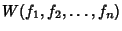. If the Wronskian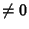for any value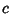in the interval, then the only solution possible for (2) is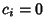(, ...,), and the functions are linearly independent. If, on the other hand,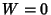for a range, the functions are linearly dependent in the range. This is equivalent to stating that if the vectors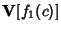, ...,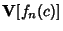defined by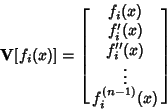(6)

are linearly independent for at least one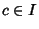, then the functions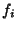are linearly independent in.

References

Sansone, G. Linearly Independent Functions.'' §1.2 in Orthogonal Functions, rev. English ed. New York: Dover, pp. 2-3, 1991.